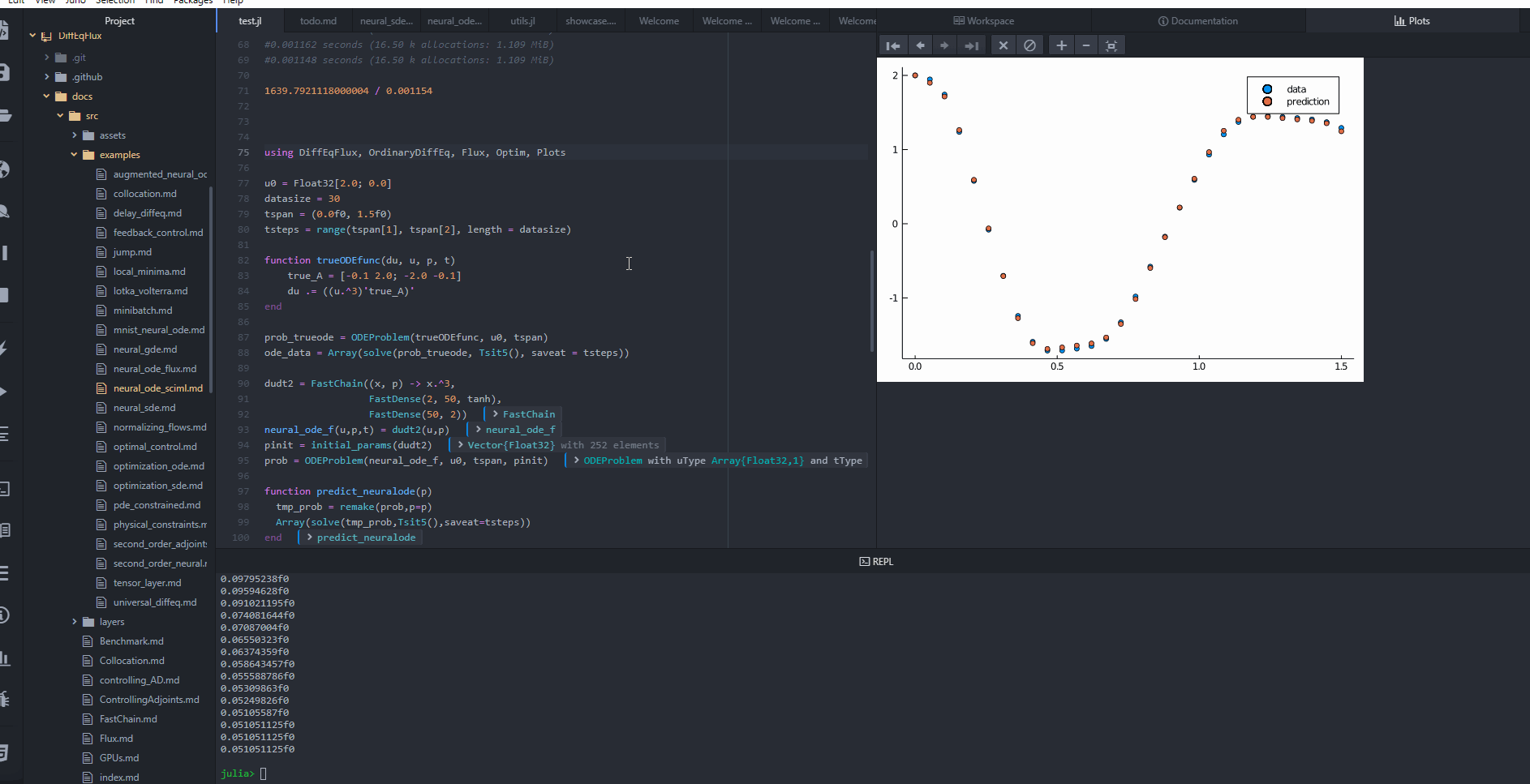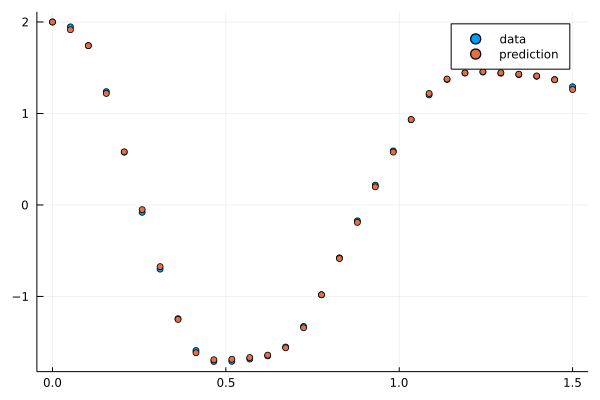# Neural Ordinary Differential Equations with sciml_train

DiffEqFlux.jl defines sciml_train which is a high level utility that automates a lot of the choices, using heuristics to determine a potentially efficient method. However, in some cases you may want more control over the optimization process. In this example we will use this utility to train a neural ODE to some generated data. A neural ODE is an ODE where a neural network defines its derivative function. Thus for example, with the multilayer perceptron neural network FastChain(FastDense(2, 50, tanh), FastDense(50, 2)), we obtain the following results.

## Copy-Pasteable Code

Before getting to the explanation, here's some code to start with. We will follow a full explanation of the definition and training process:

using DiffEqFlux, DifferentialEquations, Plots, GalacticOptim

u0 = Float32[2.0; 0.0] # Initial condition
datasize = 30 # Number of data points
tspan = (0.0f0, 1.5f0) # Time range
tsteps = range(tspan, tspan, length = datasize) # Split time range into equal steps for each data point

# Function that will generate the data we are trying to fit
function trueODEfunc(du, u, p, t)
true_A = [-0.1 2.0; -2.0 -0.1]
du .= ((u.^3)'true_A)' # Need transposes to make the matrix multiplication work
end

# Define the problem with the function above
prob_trueode = ODEProblem(trueODEfunc, u0, tspan)
# Solve and take just the solution array
ode_data = Array(solve(prob_trueode, Tsit5(), saveat = tsteps))

# Make a neural net with a NeuralODE layer
dudt2 = FastChain((x, p) -> x.^3, # Guess a cubic function
FastDense(2, 50, tanh), # Multilayer perceptron for the part we don't know
FastDense(50, 2))
prob_neuralode = NeuralODE(dudt2, tspan, Tsit5(), saveat = tsteps)

# Array of predictions from NeuralODE with parameters p starting at initial condition u0
function predict_neuralode(p)
Array(prob_neuralode(u0, p))
end

function loss_neuralode(p)
pred = predict_neuralode(p)
loss = sum(abs2, ode_data .- pred) # Just sum of squared error
return loss, pred
end

# Callback function to observe training
callback = function (p, l, pred; doplot = true)
display(l)
# plot current prediction against data
plt = scatter(tsteps, ode_data[1,:], label = "data")
scatter!(plt, tsteps, pred[1,:], label = "prediction")
if doplot
display(plot(plt))
end
return false
end

# Parameters are prob_neuralode.p
result_neuralode = DiffEqFlux.sciml_train(loss_neuralode, prob_neuralode.p,
cb = callback)## Explanation

Let's generate a time series array from a cubic equation as data:

using DiffEqFlux, DifferentialEquations, Plots

u0 = Float32[2.0; 0.0]
datasize = 30
tspan = (0.0f0, 1.5f0)
tsteps = range(tspan, tspan, length = datasize)

function trueODEfunc(du, u, p, t)
true_A = [-0.1 2.0; -2.0 -0.1]
du .= ((u.^3)'true_A)'
end

prob_trueode = ODEProblem(trueODEfunc, u0, tspan)
ode_data = Array(solve(prob_trueode, Tsit5(), saveat = tsteps))

Now let's define a neural network with a NeuralODE layer. First we define the layer. Here we're going to use FastChain, which is a faster neural network structure for NeuralODEs:

dudt2 = FastChain((x, p) -> x.^3,
FastDense(2, 50, tanh),
FastDense(50, 2))
prob_neuralode = NeuralODE(dudt2, tspan, Tsit5(), saveat = tsteps)

Note that we can directly use Chains from Flux.jl as well, for example:

dudt2 = Chain(x -> x.^3,
Dense(2, 50, tanh),
Dense(50, 2))

In our model we used the x -> x.^3 assumption in the model. By incorporating structure into our equations, we can reduce the required size and training time for the neural network, but we need a good guess!

From here, we build a loss function around our NeuralODE. NeuralODE has an optional second argument for new parameters which we will use to iteratively change the neural network in our training loop. We will use the L2 loss of the network's output against the time series data:

function predict_neuralode(p)
Array(prob_neuralode(u0, p))
end

function loss_neuralode(p)
pred = predict_neuralode(p)
loss = sum(abs2, ode_data .- pred)
return loss, pred
end

We define a callback function.

# Callback function to observe training
callback = function (p, l, pred; doplot = false)
display(l)
# plot current prediction against data
plt = scatter(tsteps, ode_data[1,:], label = "data")
scatter!(plt, tsteps, pred[1,:], label = "prediction")
if doplot
display(plot(plt))
end
return false
end

We then train the neural network to learn the ODE. sciml_train chooses heuristics that train quickly and simply:

result_neuralode = DiffEqFlux.sciml_train(loss_neuralode, prob_neuralode.p,
cb = callback)

## Usage Without the Layer Function

Note that you can equivalently define the NeuralODE by hand instead of using the NeuralODE. With FastChain this would look like:

dudt!(u, p, t) = dudt2(u, p)
u0 = rand(2)
prob = ODEProblem(dudt!, u0, tspan, p)
my_neural_ode_prob = solve(prob, Tsit5(), args...; kwargs...)

and with Chain this would look like:

p, re = Flux.destructure(dudt2)
neural_ode_f(u, p, t) = re(p)(u)
u0 = rand(2)
prob = ODEProblem(neural_ode_f, u0, tspan, p)
my_neural_ode_prob = solve(prob, Tsit5(), args...; kwargs...)

and then one would use solve for the prediction like in other tutorials.

In total, the 'from-scratch' form looks like:

using DiffEqFlux, DifferentialEquations, Plots, GalacticOptim

u0 = Float32[2.0; 0.0]
datasize = 30
tspan = (0.0f0, 1.5f0)
tsteps = range(tspan, tspan, length = datasize)

function trueODEfunc(du, u, p, t)
true_A = [-0.1 2.0; -2.0 -0.1]
du .= ((u.^3)'true_A)'
end

prob_trueode = ODEProblem(trueODEfunc, u0, tspan)
ode_data = Array(solve(prob_trueode, Tsit5(), saveat = tsteps))

dudt2 = FastChain((x, p) -> x.^3,
FastDense(2, 50, tanh),
FastDense(50, 2))
dudt!(u, p, t) = dudt2(u, p)
u0 = rand(2)
prob_neuralode = ODEProblem(dudt!, u0, tspan, initial_params(dudt2))
sol_node = solve(prob_neuralode, Tsit5(), saveat = tsteps)

function predict_neuralode(p)
tmp_prob = remake(prob_neuralode, p = p)
Array(solve(tmp_prob, Tsit5(), saveat = tsteps))
end

function loss_neuralode(p)
pred = predict_neuralode(p)
loss = sum(abs2, ode_data .- pred)
return loss, pred
end

callback = function (p, l, pred; doplot = true)
display(l)
# plot current prediction against data
plt = scatter(tsteps, ode_data[1,:], label = "data")
scatter!(plt, tsteps, pred[1,:], label = "prediction")
if doplot
display(plot(plt))
end
return false
end

result_neuralode = DiffEqFlux.sciml_train(loss_neuralode, prob_neuralode.p, cb = callback)